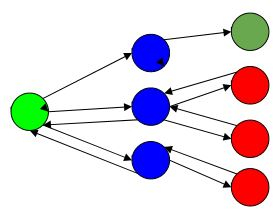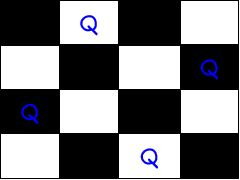# Introduction to Backtracking

Backtracking is a technique based on algorithm to solve problem. It uses recursive calling to find the solution by building a solution step by step increasing values with time. It removes the solutions that doesn't give rise to the solution of the problem based on the constraints given to solve the problem.

Backtracking algorithm is applied to some specific types of problems,

• Decision problem used to find a feasible solution of the problem.

• Optimisation problem used to find the best solution that can be applied.

• Enumeration problem used to find the set of all feasible solutions of the problem.

In backtracking problem, the algorithm tries to find a sequence path to the solution which has some small checkpoints from where the problem can backtrack if no feasible solution is found for the problem.

Example,Here,

Green is the start point, blue is the intermediate point, red are points with no feasible solution, dark green is end solution.

Here, when the algorithm propagates to an end to check if it is a solution or not, if it is then returns the solution otherwise backtracks to the point one step behind it to find track to the next point to find solution.

## Algorithm

Step 1 − if current_position is goal, return success
Step 2 − else,
Step 3 − if current_position is an end point, return failed.
Step 4 − else, if current_position is not end point, explore and repeat above steps.

Let’s use this backtracking problem to find the solution to N-Queen Problem.

In N-Queen problem, we are given an NxN chessboard and we have to place n queens on the board in such a way that no two queens attack each other. A queen will attack another queen if it is placed in horizontal, vertical or diagonal points in its way. Here, we will do 4-Queen problem.

Here, the solution is −Here, the binary output for n queen problem with 1’s as queens to the positions are placed.

{0 , 1 , 0 , 0}
{0 , 0 , 0 , 1}
{1 , 0 , 0 , 0}
{0 , 0 , 1 , 0}

For solving n queens problem, we will try placing queen into different positions of one row. And checks if it clashes with other queens. If current positioning of queens if there are any two queens attacking each other. If they are attacking, we will backtrack to previous location of the queen and change its positions. And check clash of queen again.

## Algorithm

Step 1 − Start from 1st position in the array.Step 2 − Place queens in the board and check. Do,
Step 2.1 − After placing the queen, mark the position as a part of the solution and then recursively check if this will lead to a solution.
Step 2.2 − Now, if placing the queen doesn’t lead to a solution and trackback and go to step (a) and place queens to other rows.
Step 2.3 − If placing queen returns a lead to solution return TRUE.
Step 3 − If all queens are placed return TRUE.
Step 4 − If all rows are tried and no solution is found, return FALSE.

Now, Lets use backtracking to solve the Rat in a Maze problem −

In rat in a maze problem, we are with an NxN maze the first position of the maze i.e  and will end at position [n-1][n-1] of the array. In this path there are some dead roads which do not lead to a solution.

Using backtracking in this problem we will go down step by step to reach the final goal position in the maze.

The below 2D array displays how the problem seems.Here the dashed lines show the path traveled.